markdown打公式太费劲了，所以我用手写了．（懒）　然后csdn又限制了图片文件大小，我是照完照片又截图才传上来的，所以图片有点不清晰．

# 1 高斯牛顿法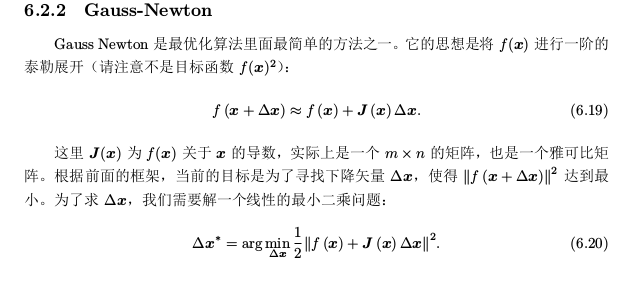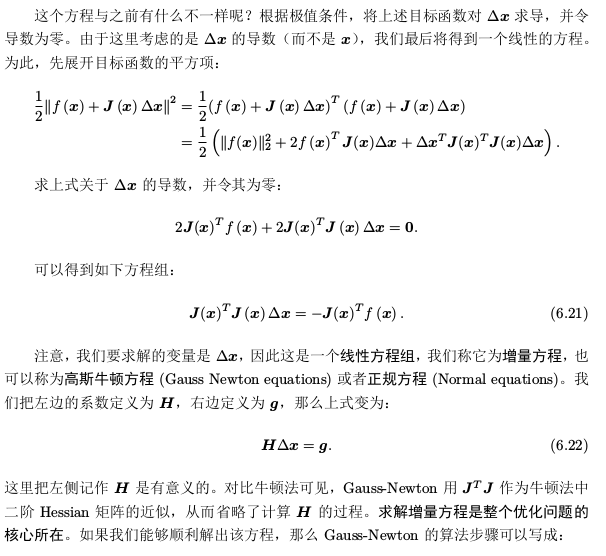# 2 公式推导

## 2.1 目标函数推导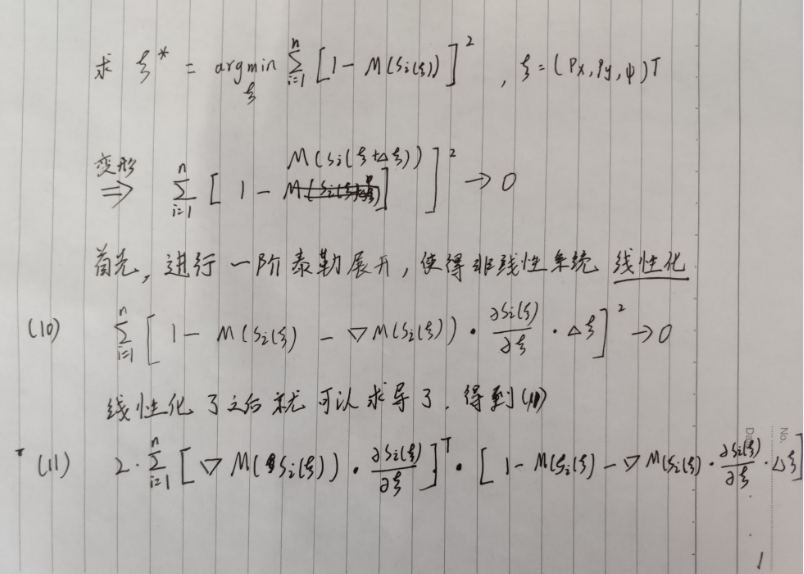之后，进行左右项的变换．求得 Δξ=H −1 ∗g．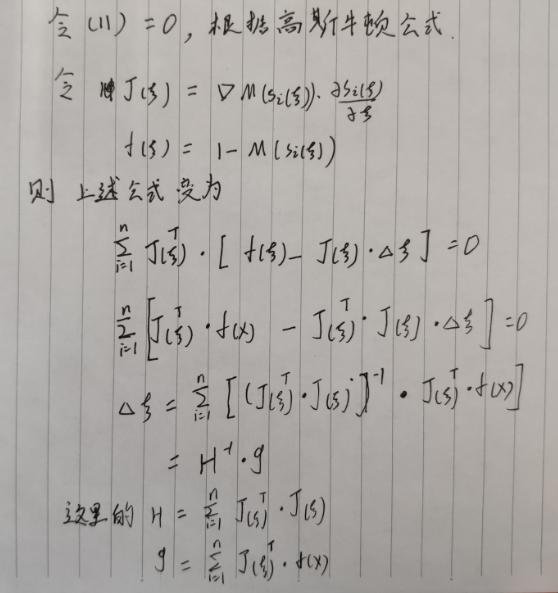## 2.2 求解

### 2.2.1 求激光点转换函数的导数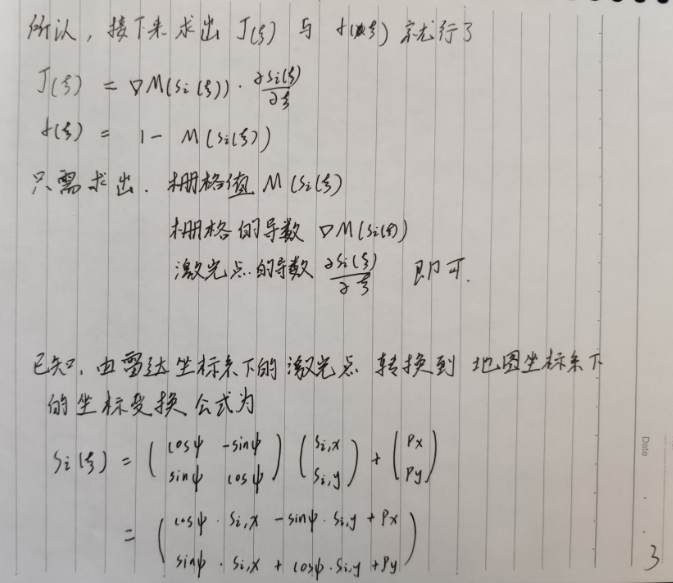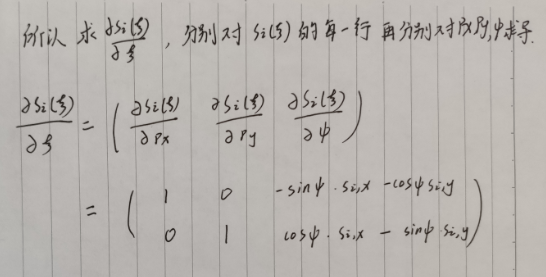### 2.2.2 求栅格值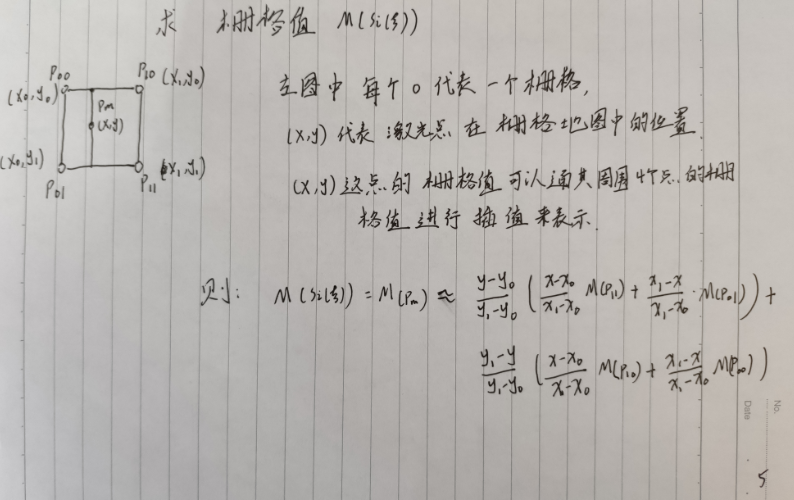### 2.2.3 求栅格值的导数 ∇M(S i (ξ))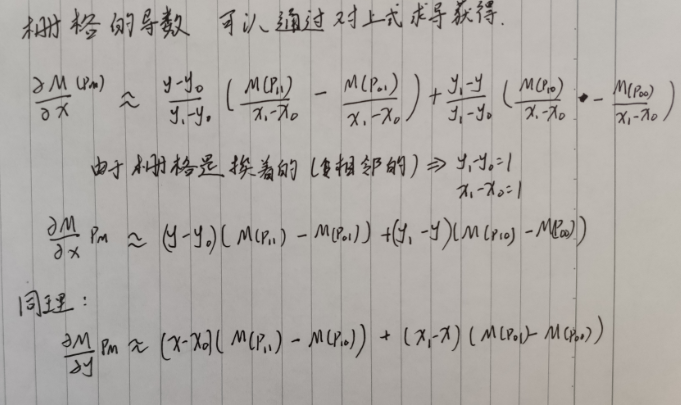### 2.2.4 求J(ξ) 与f(ξ)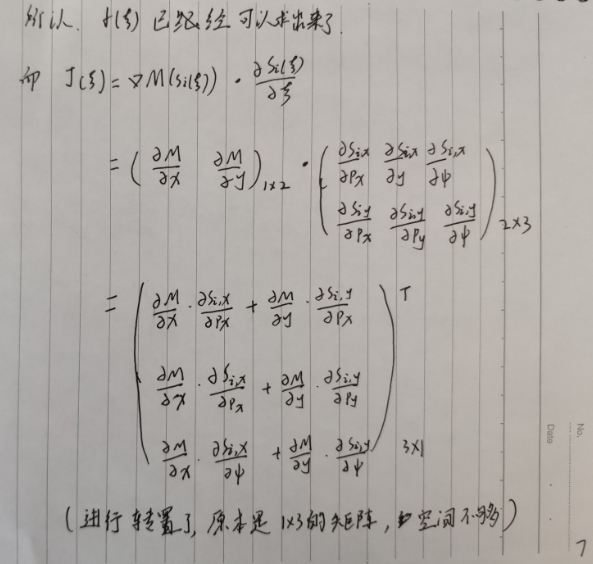### 2.2.5求Hessian矩阵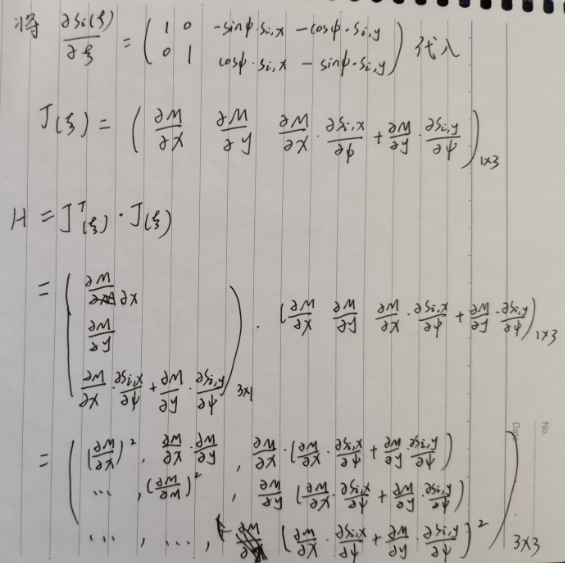# 3 相关代码讲解

## 3.1 getCompleteHessianDerivs()

    /**
* 使用当前pose投影dataPoints到地图，计算出 H 矩阵 b列向量， 理论部分详见Hector论文： 《A Flexible and Scalable SLAM System with Full 3D Motion Estimation》.
* @param pose    地图系上的位姿
* @param dataPoints  已转换为地图尺度的激光点数据
* @param H   需要计算的 H矩阵
* @param dTr  需要计算的 g列向量
*/
void getCompleteHessianDerivs(const Eigen::Vector3f &pose,
const DataContainer &dataPoints,
Eigen::Matrix3f &H,
Eigen::Vector3f &dTr)
{
int size = dataPoints.getSize();

// 获取变换矩阵
Eigen::Affine2f transform(getTransformForState(pose));

float sinRot = sin(pose);
float cosRot = cos(pose);

H = Eigen::Matrix3f::Zero();
dTr = Eigen::Vector3f::Zero();

// 按照公式计算H、b
for (int i = 0; i < size; ++i)
{
// 地图尺度下的激光坐标系下的激光点坐标
const Eigen::Vector2f &currPoint(dataPoints.getVecEntry(i));

// 将激光点坐标转换到地图系上, 通过双线性插值计算栅格概率
// transformedPointData--通过插值得到的栅格值
// transformedPointData--栅格值x方向的梯度
// transformedPointData--栅格值y方向的梯度
Eigen::Vector3f transformedPointData(interpMapValueWithDerivatives(transform * currPoint));

// 目标函数f(x)  (1-M(Pm))
float funVal = 1.0f - transformedPointData;

// 计算g列向量的 x 与 y 方向的值
dTr += transformedPointData * funVal;
dTr += transformedPointData * funVal;

// 根据公式计算
float rotDeriv = ((-sinRot * currPoint.x() - cosRot * currPoint.y()) * transformedPointData +
(cosRot * currPoint.x() - sinRot * currPoint.y()) * transformedPointData);
// 计算g列向量的 角度 的值
dTr += rotDeriv * funVal;

// 计算 hessian 矩阵
H(0, 0) += util::sqr(transformedPointData);
H(1, 1) += util::sqr(transformedPointData);
H(2, 2) += util::sqr(rotDeriv);

H(0, 1) += transformedPointData * transformedPointData;
H(0, 2) += transformedPointData * rotDeriv;
H(1, 2) += transformedPointData * rotDeriv;
}

// H是对称矩阵，只算上三角就行， 减少计算量。
H(1, 0) = H(0, 1);
H(2, 0) = H(0, 2);
H(2, 1) = H(1, 2);
}


## 3.2 interpMapValueWithDerivatives()

    /**
* 双线性插值计算网格中任一点的得分（占据概率）以及该点处的梯度
* @param coords  激光点地图坐标
* @return ret(0) 是网格值 ， ret(1) 是栅格值在x方向的导数 ， ret(2)是栅格值在y方向的导数
*/
Eigen::Vector3f interpMapValueWithDerivatives(const Eigen::Vector2f &coords)
{
// 检查coords坐标是否是在地图坐标范围内
if (concreteGridMap->pointOutOfMapBounds(coords))
{
return Eigen::Vector3f(0.0f, 0.0f, 0.0f);
}

// 对坐标进行向下取整，即得到坐标(x0,y0)
Eigen::Vector2i indMin(coords.cast<int>());

// 得到双线性插值的因子
// | x |   | x0 |   | x-x0 |
// |   | - |    | = |      |
// | y |   | y0 |   | y-y0 |
Eigen::Vector2f factors(coords - indMin.cast<float>());

// 获得地图的X方向最大边界
int sizeX = concreteGridMap->getSizeX();

// 计算(x0, y0)点的网格索引值
int index = indMin * sizeX + indMin;

// 下边这取4个点的栅格值，感觉就是导致hector大地图后计算变慢的原因

/** 首先判断cache中该地图点在本次scan中是否被访问过，若有则直接取值；没有则立马计算概率值并更新到cache **/
/** 这个cache的作用是，避免单次scan重复访问同一网格时带来的重复概率计算。地图更新后，网格logocc改变，cache数据就会无效。 **/
/** 但是这种方式内存开销太大..相当于同时维护两份地图，使用 hash map 是不是会更合适些 **/
if (!cacheMethod.containsCachedData(index, intensities))
{
intensities = getUnfilteredGridPoint(index); // 得到M(P00),P00(x0,y0)
cacheMethod.cacheData(index, intensities);
}

++index;
if (!cacheMethod.containsCachedData(index, intensities))
{
intensities = getUnfilteredGridPoint(index);
cacheMethod.cacheData(index, intensities);
}

index += sizeX - 1;
if (!cacheMethod.containsCachedData(index, intensities))
{
intensities = getUnfilteredGridPoint(index);
cacheMethod.cacheData(index, intensities);
}

++index;
if (!cacheMethod.containsCachedData(index, intensities))
{
intensities = getUnfilteredGridPoint(index);
cacheMethod.cacheData(index, intensities);
}

float dx1 = intensities - intensities; // 求得(M(P00) - M(P10))的值
float dx2 = intensities - intensities; // 求得(M(P01) - M(P11))的值

float dy1 = intensities - intensities; // 求得(M(P00) - M(P01))的值
float dy2 = intensities - intensities; // 求得(M(P10) - M(P11))的值

// 得到双线性插值的因子,注意x0+1=x1,y0+1=y1,则
//     | x-x0 |   | 1-x+x0 |   | x1-x |
// 1 - |      | = |        | = |      |
//     | y-y0 |   | 1-y+y0 |   | y1-x |
float xFacInv = (1.0f - factors); // 求得(x1-x)的值
float yFacInv = (1.0f - factors); // 求得(y1-y)的值

//         y-y0 | x-x0            x1-x        |    y1-y | x-x0            x1-x        |
// M(Pm) = ------|------ M(P11) + ------ M(P01)| + ------|------ M(P10) + ------ M(P00)|
//         y1-y0| x1-x0           x1-x0       |    y1-y0| x1-x0           x1-x0       |
// 注意：此处y1-y0=x1-x0=1,那么对应函数返回值，可以写成
// M(Pm) = (M(P00) * (x1-x) + M(P10) * (x-x0)) * (y1-y) + (M(P01) * (x1-x) + M(P11) * (x-x0)) * (y-y0)

// d(M(Pm))     y-y0 |                |    y1-y |                |
// ---------- = ------| M(P11) - M(P01)| + ------| M(P10) - M(P00)|
//    dx        y1-y0|                |    y1-y0|                |
// 同理，化简可得 d(M(Pm))/dx = -((M(P00) - M(P10)) * (y1-y) + (M(P01) - M(P11)) * (y-y0))
// 同样地，也有   d(M(Pm))/dy = -((M(P00) - M(P01)) * (x1-x) + (M(P10) - M(P11)) * (x-x0))

// 计算网格值，计算梯度 --- 原版这里的dx、dy的计算有错误，已经改过来了
return Eigen::Vector3f(
((intensities * xFacInv + intensities * factors) * (yFacInv)) +
((intensities * xFacInv + intensities * factors) * (factors)),
-((dx1 * yFacInv) + (dx2 * factors)),
-((dy1 * xFacInv) + (dy2 * factors))
// -((dx1 * xFacInv) + (dx2 * factors)), // 应该为： -((dx1 * yFacInv) + (dx2 * factors))
// -((dy1 * yFacInv) + (dy2 * factors))  // 应该为： -((dy1 * xFacInv) + (dy2 * factors))
);
}


# 5 总结与思考

## 5.1 总结

• 首先就是hector的计算太慢了，只进行扫描匹配的操作就要花费大概0.1秒钟，这就导致即使雷达频率再高，hector也处理不过来
• 还有就是hector的地图不能自动更改大小，地图的大小在初始化之后始终是固定的
• hector中获取栅格点时使用了cache，这块实现的不是特别好
• hector中维护的始终是一张地图，有时会出现地图被错误更新之后再也恢复不了的情况

## 5.2 思考

gmapping与hector维护地图的方式不同.

gmapping的维护地图是通过保存下来的位姿与相应的雷达数据，每次发布的地图都是新生成的地图，将所有的保存的雷达数据写成地图。

hector的维护地图是在初始化阶段时只初始化一张地图，然后使用新的雷达数据对这张地图进行更新与维护。

# 6 NEXT

hector其实还可以做很多改进，如将里程计或者imu加进来做位姿预测，地图大小可以动态更新，计算地图这可以使用ceres库等等。

github上有人对hector的高斯牛顿这的代码做了改进，使用ceres库进行计算，感兴趣的可以在github上搜索 hector_slam_Ceres 查看代码。